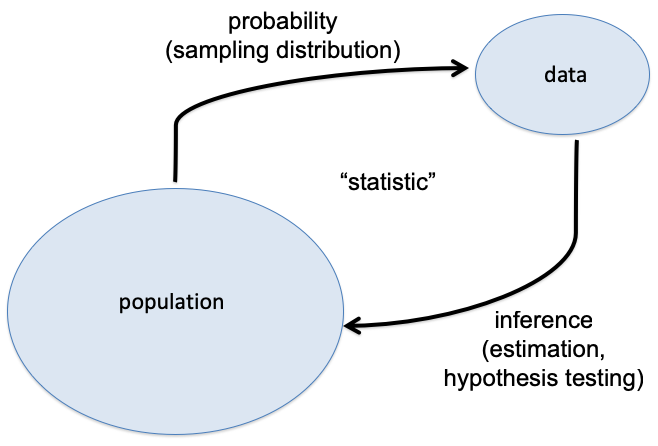# 10 Probability and Statistics

Probabilistic modeling and/or statistical inference are required when the goals include:

1. Characterizing randomness or “noise” in the data
2. Quantifying uncertainty in models we build or decisions we make from the data
3. Predicting future observations or decisions in the face of uncertainty

## 10.1 Central Dogma of InferenceFigure 10.1: Central Dogma of Statistical Inference

## 10.2 Data Analysis Without Probability

It is possible to do data analysis without probability and formal statistical inference:

• Descriptive statistics can be reported without utilizing probability and statistical inference
• Exploratory data analysis and visualization tend to not involve probability or formal statistical inference
• Important problems in machine learning do not involve probability or statistical inference.